# Solution to Rubik's Clock

The goal of this manual is to show the reader how to solve Rubik's Clock. Note that it is not the intention to explain how it works. For example, details about what clocks rotate under which circumstances are left out.

To solve Rubik's Clock, perform the following steps:

1. You first need to know how to solve a row of three clocks. Let's say the initial situation is the following: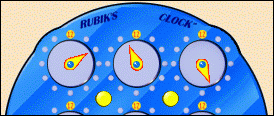To solve this row, do the following;
• Make sure all four yellow buttons are pressed, except for upper left one. This is shown in the following picture: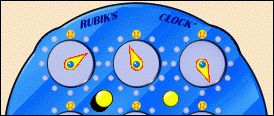• Turn the upper left wheel until the second clock equals the third one.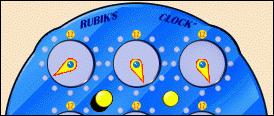• Pull the upper right button, like this: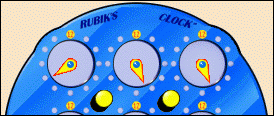• Turn the upper right wheel until the first clock reaches twelve o'clock.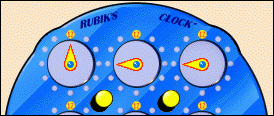• Press the upper left button.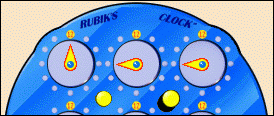• Turn the upper right wheel until the second and third clock both reach twelve o'clock. Congratulations, you have solved a row.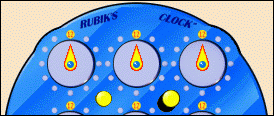2. If you properly executed the previous step, you now have solved clocks numbered 1 to 3, according to the following numbering:
 1 2 3 4 5 6 7 8 9
To complete the whole front side, do the following:
• To solve clocks 3, 6 and 9, rotate the whole box 90 degrees counter clockwise, and repeat step 1.
• Rotate the whole box another 90 degrees counter clockwise, and repeat step 1. This solves clocks 9, 8 and 7.
• Rotate the whole box another 90 degrees counter clockwise, and repeat step 1. This solves clocks 7, 4 and 1.
• Rotate the whole box 135 (90+45) degrees counter clockwise. Clocks 1, 5 and 9 now should be on a straight horizontal line. Now repeat step 1. You should be able to figure out what buttons to press by now!
You now have completed one side of the box!
3. Turn the box around, and repeat steps 1 and 2. After doing so, you should have solved Rubik's Clock.